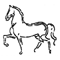### Generic Module: Red Typhoon II Journal

 Number of entries per page: 20 50 100 ALL Sort order: Most recent entries first Oldest entries first

Filter on: (filter will search Player, Title, and Entry)

View elapsed game time

 Entry # Time Turn Player Title Entry 4 9/6/2021 7:54:00 PM Turn 1 Soviets Message I'll update the combat log, and update the Vassal board tomorrow morning. 3 9/6/2021 7:53:00 PM Turn 1 Soviets Die roll request Request: 6-sided die x 1145644363223Message from Soviets:1) Hexes 1706+1806+1807 vs 1707 = 18:3 = 6:1 with -1 drm (woods) 2) Hexes 2109+2309+2310 vs 2209 = 20:5 = 4:1 with -1 drm (woods) 3) Hexes 2510+2610 vs 2511 = 15:5 = 3:1 with -1 drm (woods) 4) Hexes 3115+3215 vs 3116 = 16:4 = 4:1 with -1 drm (woods) 5) Hexes 3216+3317+3318 vs 3217 = 29:7 = 4:1 6) Hexes 3418+3519+3520 vs 3419 = 22:7 = 3:1 7) Hexes 3526+3527+3427 vs 3426 = 24:8 = 3:1 with -1 drm (woods) 8) Hexes 3328+3229 vs 3228 = 12:4 = 3:1 9) Hexes 3130+2930 vs 3029 = 17:4 = 4:1 with -1 drm (woods) 10) Hexes 2630+2531 vs 2530 = 15:3 = 5:1 with -1 drm (woods) 11) Hexes 2431+2333 vs 2332 = 12:3 = 4:1 with -1 drm (river) 2 8/17/2021 10:49:00 AM Turn 1 Soviets Die roll request Request: 6-sided die x 9342563461Message from Soviets:Soviet Turn 2 Combat:1) Hexes 2004+2105+2106 vs 2005 = 18:3 = 6:1 with -1 drm (woods) 2) Hexes 2107 and 2208 vs.2108 = 16:4 = 4:1 with -1 drm (woods)3) Hexes 2810+2911+2912 vs.2811 = 20:4 = 5:1 with -1 drm (woods)4) Hexes 3315+3415+3416 vs. 3316 =21:4 = 5:1 with -1 drm (woods)5) Hexes 3417+3418 vs 3318 = 27:9 = 3:1 with no drm.6) Hexes 3521+3621+3622 vs. 3522 = 30:10 = 3:1 with -1 drm (woods)7) Hexes 3528+3529+3429 vs. 3428 = 20:4 = 5:1 with -1 drm (woods)8) Hexes 3030+2931 vs.2930 = 15:3 = 5:1 with -1 drm (woods)9) Hexes 2932+2933+2733 vs.2832 = 20:4 = 5:1 with no drm (woods, isolation) 1 7/25/2021 4:46:00 PM Turn 1 Soviets Die roll request Request: 6-sided die x 103264261165Message from Soviets:Turn 1 Soviet Attacks:1) Hex 2502 is 6:1 with +0 net drm2) Hex 2403 is 4:1 with +2 net drm3) Hex 2308 is 3:1 with +0 net drm4) Hex 2509 is 3:1 with +0 net drm. 5) Hex 3013 is 4:1 with +1 net drm. 6) Hex 3214 is 5:1 with +1 net drm.7) Hex 3418 is 3:1 with +2 net drm.8) Hex 3521 is 3:1 with +1 drm 9) Hex 3527 is 2:1 with +1 net drm.10) Hex 3130 is 3:1 with +2 drm.

Next 100 entries